Elementary Algebra 2e

# 7.3Factor Trinomials of the Form ax2+bx+c

Elementary Algebra 2e7.3 Factor Trinomials of the Form ax2+bx+c

### Learning Objectives

By the end of this section, you will be able to:

• Recognize a preliminary strategy to factor polynomials completely
• Factor trinomials of the form $ax2+bx+cax2+bx+c$ with a GCF
• Factor trinomials using trial and error
• Factor trinomials using the ‘ac’ method

### Be Prepared 7.8

Before you get started, take this readiness quiz.

Find the GCF of $45p2and30p645p2and30p6$.
If you missed this problem, review Example 7.2.

### Be Prepared 7.9

Multiply $(3y+4)(2y+5)(3y+4)(2y+5)$.
If you missed this problem, review Example 6.40.

### Be Prepared 7.10

Combine like terms $12x2+3x+5x+912x2+3x+5x+9$.
If you missed this problem, review Example 1.24.

### Recognize a Preliminary Strategy for Factoring

Let’s summarize where we are so far with factoring polynomials. In the first two sections of this chapter, we used three methods of factoring: factoring the GCF, factoring by grouping, and factoring a trinomial by “undoing” FOIL. More methods will follow as you continue in this chapter, as well as later in your studies of algebra.

How will you know when to use each factoring method? As you learn more methods of factoring, how will you know when to apply each method and not get them confused? It will help to organize the factoring methods into a strategy that can guide you to use the correct method.

As you start to factor a polynomial, always ask first, “Is there a greatest common factor?” If there is, factor it first.

The next thing to consider is the type of polynomial. How many terms does it have? Is it a binomial? A trinomial? Or does it have more than three terms?

If it is a trinomial where the leading coefficient is one, $x2+bx+cx2+bx+c$, use the “undo FOIL” method.

If it has more than three terms, try the grouping method. This is the only method to use for polynomials of more than three terms.

Some polynomials cannot be factored. They are called “prime.”

Below we summarize the methods we have so far. These are detailed in Choose a strategy to factor polynomials completely.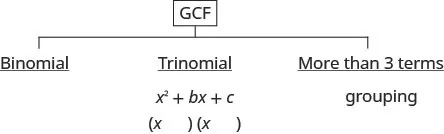### How To

#### Choose a strategy to factor polynomials completely.

1. Step 1.
Is there a greatest common factor?
• Factor it out.
2. Step 2.
Is the polynomial a binomial, trinomial, or are there more than three terms?
• If it is a binomial, right now we have no method to factor it.
• If it is a trinomial of the form $x2+bx+cx2+bx+c$: Undo FOIL $(x)(x)(x)(x)$
• If it has more than three terms: Use the grouping method.
3. Step 3. Check by multiplying the factors.

Use the preliminary strategy to completely factor a polynomial. A polynomial is factored completely if, other than monomials, all of its factors are prime.

### Example 7.29

Identify the best method to use to factor each polynomial.

1. $6y2−726y2−72$
2. $r2−10r−24r2−10r−24$
3. $p2+5p+pq+5qp2+5p+pq+5q$

### Try It 7.57

Identify the best method to use to factor each polynomial:

1. $4y2+324y2+32$
2. $y2+10y+21y2+10y+21$
3. $yz+2y+3z+6yz+2y+3z+6$

### Try It 7.58

Identify the best method to use to factor each polynomial:

1. $ab+a+4b+4ab+a+4b+4$
2. $3k2+153k2+15$
3. $p2+9p+8p2+9p+8$

### Factor Trinomials of the form ax2 + bx + c with a GCF

Now that we have organized what we’ve covered so far, we are ready to factor trinomials whose leading coefficient is not 1, trinomials of the form $ax2+bx+cax2+bx+c$.

Remember to always check for a GCF first! Sometimes, after you factor the GCF, the leading coefficient of the trinomial becomes 1 and you can factor it by the methods in the last section. Let’s do a few examples to see how this works.

Watch out for the signs in the next two examples.

### Example 7.30

Factor completely: $2n2−8n−422n2−8n−42$.

### Try It 7.59

Factor completely: $4m2−4m−84m2−4m−8$.

### Try It 7.60

Factor completely: $5k2−15k−505k2−15k−50$.

### Example 7.31

Factor completely: $4y2−36y+564y2−36y+56$.

### Try It 7.61

Factor completely: $3r2−9r+63r2−9r+6$.

### Try It 7.62

Factor completely: $2t2−10t+122t2−10t+12$.

In the next example the GCF will include a variable.

### Example 7.32

Factor completely: $4u3+16u2−20u4u3+16u2−20u$.

### Try It 7.63

Factor completely: $5x3+15x2−20x5x3+15x2−20x$.

### Try It 7.64

Factor completely: $6y3+18y2−60y6y3+18y2−60y$.

### Factor Trinomials using Trial and Error

What happens when the leading coefficient is not 1 and there is no GCF? There are several methods that can be used to factor these trinomials. First we will use the Trial and Error method.

Let’s factor the trinomial $3x2+5x+23x2+5x+2$.

From our earlier work we expect this will factor into two binomials.

$3x2+5x+2()()3x2+5x+2()()$

We know the first terms of the binomial factors will multiply to give us $3x23x2$. The only factors of $3x23x2$ are $1x,3x1x,3x$. We can place them in the binomials.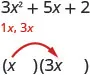Check. Does $1x·3x=3x21x·3x=3x2$?

We know the last terms of the binomials will multiply to 2. Since this trinomial has all positive terms, we only need to consider positive factors. The only factors of 2 are 1 and 2. But we now have two cases to consider as it will make a difference if we write 1, 2, or 2, 1.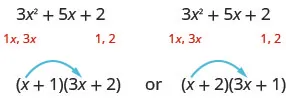Which factors are correct? To decide that, we multiply the inner and outer terms.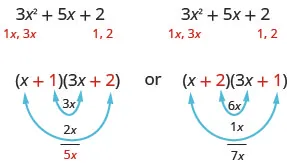Since the middle term of the trinomial is 5x, the factors in the first case will work. Let’s FOIL to check.

$(x+1)(3x+2)3x2+2x+3x+23x2+5x+2✓(x+1)(3x+2)3x2+2x+3x+23x2+5x+2✓$

Our result of the factoring is:

$3x2+5x+2(x+1)(3x+2)3x2+5x+2(x+1)(3x+2)$

### Example 7.33

#### How to Factor Trinomials of the Form $ax2+bx+cax2+bx+c$ Using Trial and Error

Factor completely: $3y2+22y+73y2+22y+7$.

### Try It 7.65

Factor completely: $2a2+5a+32a2+5a+3$.

### Try It 7.66

Factor completely: $4b2+5b+14b2+5b+1$.

### How To

#### Factor trinomials of the form $ax2+bx+cax2+bx+c$ using trial and error.

1. Step 1. Write the trinomial in descending order of degrees.
2. Step 2. Find all the factor pairs of the first term.
3. Step 3. Find all the factor pairs of the third term.
4. Step 4. Test all the possible combinations of the factors until the correct product is found.
5. Step 5. Check by multiplying.

When the middle term is negative and the last term is positive, the signs in the binomials must both be negative.

### Example 7.34

Factor completely: $6b2−13b+56b2−13b+5$.

### Try It 7.67

Factor completely: $8x2−14x+38x2−14x+3$.

### Try It 7.68

Factor completely: $10y2−37y+710y2−37y+7$.

When we factor an expression, we always look for a greatest common factor first. If the expression does not have a greatest common factor, there cannot be one in its factors either. This may help us eliminate some of the possible factor combinations.

### Example 7.35

Factor completely: $14x2−47x−714x2−47x−7$.

### Try It 7.69

Factor completely: $8a2−3a−58a2−3a−5$.

### Try It 7.70

Factor completely: $6b2−b−156b2−b−15$.

### Example 7.36

Factor completely: $18n2−37n+1518n2−37n+15$.

### Try It 7.71

Factor completely: $18x2−3x−1018x2−3x−10$.

### Try It 7.72

Factor completely: $30y2−53y−2130y2−53y−21$.

Don’t forget to look for a GCF first.

### Example 7.37

Factor completely: $10y4+55y3+60y210y4+55y3+60y2$.

### Try It 7.73

Factor completely: $15n3−85n2+100n15n3−85n2+100n$.

### Try It 7.74

Factor completely: $56q3+320q2−96q56q3+320q2−96q$.

### Factor Trinomials using the “ac” Method

Another way to factor trinomials of the form $ax2+bx+cax2+bx+c$ is the “ac” method. (The “ac” method is sometimes called the grouping method.) The “ac” method is actually an extension of the methods you used in the last section to factor trinomials with leading coefficient one. This method is very structured (that is step-by-step), and it always works!

### Example 7.38

#### How to Factor Trinomials Using the “ac” Method

Factor: $6x2+7x+26x2+7x+2$.

### Try It 7.75

Factor: $6x2+13x+26x2+13x+2$.

### Try It 7.76

Factor: $4y2+8y+34y2+8y+3$.

### How To

#### Factor trinomials of the form using the “ac” method.

1. Step 1. Factor any GCF.
2. Step 2. Find the product ac.
3. Step 3. Find two numbers m and n that:
$Multiply toacm·n=a·cAdd tobm+n=bMultiply toacm·n=a·cAdd tobm+n=b$
4. Step 4. Split the middle term using m and n: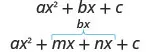5. Step 5. Factor by grouping.
6. Step 6. Check by multiplying the factors.

When the third term of the trinomial is negative, the factors of the third term will have opposite signs.

### Example 7.39

Factor: $8u2−17u−218u2−17u−21$.

### Try It 7.77

Factor: $20h2+13h−1520h2+13h−15$.

### Try It 7.78

Factor: $6g2+19g−206g2+19g−20$.

### Example 7.40

Factor: $2x2+6x+52x2+6x+5$.

### Try It 7.79

Factor: $10t2+19t−1510t2+19t−15$.

### Try It 7.80

Factor: $3u2+8u+53u2+8u+5$.

Don’t forget to look for a common factor!

### Example 7.41

Factor: $10y2−55y+7010y2−55y+70$.

### Try It 7.81

Factor: $16x2−32x+1216x2−32x+12$.

### Try It 7.82

Factor: $18w2−39w+1818w2−39w+18$.

We can now update the Preliminary Factoring Strategy, as shown in Figure 7.2 and detailed in Choose a strategy to factor polynomials completely (updated), to include trinomials of the form $ax2+bx+cax2+bx+c$. Remember, some polynomials are prime and so they cannot be factored.

Figure 7.2

### How To

#### Choose a strategy to factor polynomials completely (updated).

1. Step 1.
Is there a greatest common factor?
• Factor it.
2. Step 2.
Is the polynomial a binomial, trinomial, or are there more than three terms?
• If it is a binomial, right now we have no method to factor it.
• If it is a trinomial of the form $x2+bx+cx2+bx+c$
Undo FOIL $(x)(x)(x)(x)$.
• If it is a trinomial of the form $ax2+bx+cax2+bx+c$
Use Trial and Error or the “ac” method.
• If it has more than three terms
Use the grouping method.
3. Step 3. Check by multiplying the factors.

### Media

Access these online resources for additional instruction and practice with factoring trinomials of the form $ax2+bx+cax2+bx+c$.

### Section 7.3 Exercises

#### Practice Makes Perfect

Recognize a Preliminary Strategy to Factor Polynomials Completely

In the following exercises, identify the best method to use to factor each polynomial.

135.
1. $10q2+5010q2+50$
2. $a2−5a−14a2−5a−14$
3. $uv+2u+3v+6uv+2u+3v+6$
136.
1. $n2+10n+24n2+10n+24$
2. $8u2+168u2+16$
3. $pq+5p+2q+10pq+5p+2q+10$
137.
1. $x2+4x−21x2+4x−21$
2. $ab+10b+4a+40ab+10b+4a+40$
3. $6c2+246c2+24$
138.
1. $20x2+10020x2+100$
2. $uv+6u+4v+24uv+6u+4v+24$
3. $y2−8y+15y2−8y+15$

Factor Trinomials of the form $ax2+bx+cax2+bx+c$ with a GCF

In the following exercises, factor completely.

139.

$5 x 2 + 35 x + 30 5 x 2 + 35 x + 30$

140.

$12 s 2 + 24 s + 12 12 s 2 + 24 s + 12$

141.

$2 z 2 − 2 z − 24 2 z 2 − 2 z − 24$

142.

$3 u 2 − 12 u − 36 3 u 2 − 12 u − 36$

143.

$7 v 2 − 63 v + 56 7 v 2 − 63 v + 56$

144.

$5 w 2 − 30 w + 45 5 w 2 − 30 w + 45$

145.

$p 3 − 8 p 2 − 20 p p 3 − 8 p 2 − 20 p$

146.

$q 3 − 5 q 2 − 24 q q 3 − 5 q 2 − 24 q$

147.

$3 m 3 − 21 m 2 + 30 m 3 m 3 − 21 m 2 + 30 m$

148.

$11 n 3 − 55 n 2 + 44 n 11 n 3 − 55 n 2 + 44 n$

149.

$5 x 4 + 10 x 3 − 75 x 2 5 x 4 + 10 x 3 − 75 x 2$

150.

$6 y 4 + 12 y 3 − 48 y 2 6 y 4 + 12 y 3 − 48 y 2$

Factor Trinomials Using Trial and Error

In the following exercises, factor.

151.

$2 t 2 + 7 t + 5 2 t 2 + 7 t + 5$

152.

$5 y 2 + 16 y + 11 5 y 2 + 16 y + 11$

153.

$11 x 2 + 34 x + 3 11 x 2 + 34 x + 3$

154.

$7 b 2 + 50 b + 7 7 b 2 + 50 b + 7$

155.

$4 w 2 − 5 w + 1 4 w 2 − 5 w + 1$

156.

$5 x 2 − 17 x + 6 5 x 2 − 17 x + 6$

157.

$6 p 2 − 19 p + 10 6 p 2 − 19 p + 10$

158.

$21 m 2 − 29 m + 10 21 m 2 − 29 m + 10$

159.

$4 q 2 − 7 q − 2 4 q 2 − 7 q − 2$

160.

$10 y 2 − 53 y − 11 10 y 2 − 53 y − 11$

161.

$4 p 2 + 17 p − 15 4 p 2 + 17 p − 15$

162.

$6 u 2 + 5 u − 14 6 u 2 + 5 u − 14$

163.

$16 x 2 − 32 x + 16 16 x 2 − 32 x + 16$

164.

$81 a 2 + 153 a − 18 81 a 2 + 153 a − 18$

165.

$30 q 3 + 140 q 2 + 80 q 30 q 3 + 140 q 2 + 80 q$

166.

$5 y 3 + 30 y 2 − 35 y 5 y 3 + 30 y 2 − 35 y$

Factor Trinomials using the ‘ac’ Method

In the following exercises, factor.

167.

$5 n 2 + 21 n + 4 5 n 2 + 21 n + 4$

168.

$8 w 2 + 25 w + 3 8 w 2 + 25 w + 3$

169.

$9 z 2 + 15 z + 4 9 z 2 + 15 z + 4$

170.

$3 m 2 + 26 m + 48 3 m 2 + 26 m + 48$

171.

$4 k 2 − 16 k + 15 4 k 2 − 16 k + 15$

172.

$4 q 2 − 9 q + 5 4 q 2 − 9 q + 5$

173.

$5 s 2 − 9 s + 4 5 s 2 − 9 s + 4$

174.

$4 r 2 − 20 r + 25 4 r 2 − 20 r + 25$

175.

$6 y 2 + y − 15 6 y 2 + y − 15$

176.

$6 p 2 + p − 22 6 p 2 + p − 22$

177.

$2 n 2 − 27 n − 45 2 n 2 − 27 n − 45$

178.

$12 z 2 − 41 z − 11 12 z 2 − 41 z − 11$

179.

$3 x 2 + 5 x + 4 3 x 2 + 5 x + 4$

180.

$4 y 2 + 15 y + 6 4 y 2 + 15 y + 6$

181.

$60 y 2 + 290 y − 50 60 y 2 + 290 y − 50$

182.

$6 u 2 − 46 u − 16 6 u 2 − 46 u − 16$

183.

$48 z 3 − 102 z 2 − 45 z 48 z 3 − 102 z 2 − 45 z$

184.

$90 n 3 + 42 n 2 − 216 n 90 n 3 + 42 n 2 − 216 n$

185.

$16 s 2 + 40 s + 24 16 s 2 + 40 s + 24$

186.

$24 p 2 + 160 p + 96 24 p 2 + 160 p + 96$

187.

$48 y 2 + 12 y − 36 48 y 2 + 12 y − 36$

188.

$30 x 2 + 105 x − 60 30 x 2 + 105 x − 60$

Mixed Practice

In the following exercises, factor.

189.

$12 y 2 − 29 y + 14 12 y 2 − 29 y + 14$

190.

$12 x 2 + 36 y − 24 z 12 x 2 + 36 y − 24 z$

191.

$a 2 − a − 20 a 2 − a − 20$

192.

$m 2 − m − 12 m 2 − m − 12$

193.

$6 n 2 + 5 n − 4 6 n 2 + 5 n − 4$

194.

$12 y 2 − 37 y + 21 12 y 2 − 37 y + 21$

195.

$2 p 2 + 4 p + 3 2 p 2 + 4 p + 3$

196.

$3 q 2 + 6 q + 2 3 q 2 + 6 q + 2$

197.

$13 z 2 + 39 z − 26 13 z 2 + 39 z − 26$

198.

$5 r 2 + 25 r + 30 5 r 2 + 25 r + 30$

199.

$x 2 + 3 x − 28 x 2 + 3 x − 28$

200.

$6 u 2 + 7 u − 5 6 u 2 + 7 u − 5$

201.

$3 p 2 + 21 p 3 p 2 + 21 p$

202.

$7 x 2 − 21 x 7 x 2 − 21 x$

203.

$6 r 2 + 30 r + 36 6 r 2 + 30 r + 36$

204.

$18 m 2 + 15 m + 3 18 m 2 + 15 m + 3$

205.

$24 n 2 + 20 n + 4 24 n 2 + 20 n + 4$

206.

$4 a 2 + 5 a + 2 4 a 2 + 5 a + 2$

207.

$x 2 + 2 x − 24 x 2 + 2 x − 24$

208.

$2 b 2 − 7 b + 4 2 b 2 − 7 b + 4$

#### Everyday Math

209.

Height of a toy rocket The height of a toy rocket launched with an initial speed of 80 feet per second from the balcony of an apartment building is related to the number of seconds, t, since it is launched by the trinomial $−16t2+80t+96−16t2+80t+96$. Completely factor the trinomial.

210.

Height of a beach ball The height of a beach ball tossed up with an initial speed of 12 feet per second from a height of 4 feet is related to the number of seconds, t, since it is tossed by the trinomial $−16t2+12t+4−16t2+12t+4$. Completely factor the trinomial.

#### Writing Exercises

211.

List, in order, all the steps you take when using the “ac” method to factor a trinomial of the form $ax2+bx+c.ax2+bx+c.$

212.

How is the “ac” method similar to the “undo FOIL” method? How is it different?

213.

What are the questions, in order, that you ask yourself as you start to factor a polynomial? What do you need to do as a result of the answer to each question?

214.

On your paper draw the chart that summarizes the factoring strategy. Try to do it without looking at the book. When you are done, look back at the book to finish it or verify it.

#### Self Check

After completing the exercises, use this checklist to evaluate your mastery of the objectives of this section.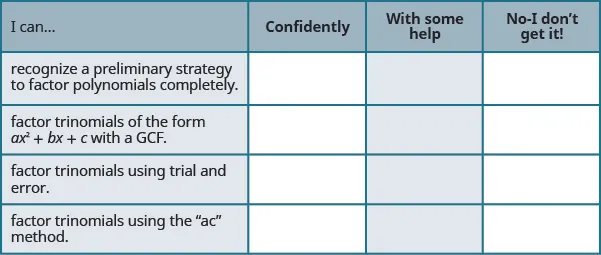What does this checklist tell you about your mastery of this section? What steps will you take to improve?

Order a print copy

As an Amazon Associate we earn from qualifying purchases.